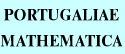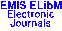PORTUGALIAEMATHEMATICA Vol. 56, No. 3, pp. 299-308 (1999)

Previous Article

Next Article

Contents of this Issue

Other Issues

ELibM Journals

ELibM Home

EMIS Home

## On Nonhomogeneous Biharmonic Equations Involving Critical Sobolev Exponent

### M. Guedda

LAMFA, Faculté de Mathématiques et d'Informatique, Université de Picardie Jules Verne,
33, rue Saint-Leu, 80039 Amiens - FRANCE

Abstract: In this paper we consider the problem $\Delta^2 u=\lambda\,|u|^{q_c-2}\,u+f$ in $\Omega$, $u=\Delta u=0$ on $\partial\Omega$, where ${q_c}=2N/(N-4)$, $N>4$, is the limiting Sobolev exponent and $\Omega$ is a smooth bounded domain in ${\R}^N$. Under some restrictions on $f$ and $\lambda$, the existence of weak solution $u$ is proved. Moreover $u\geq0$ for $f\geq 0$ whenever $\lambda\geq 0$.

Classification (MSC2000): 35J65, 35J20, 49J45.

Full text of the article: